### Home > A2C > Chapter 10 > Lesson 10.1.1 > Problem10-13

10-13.
1. Rondal High School had a student enrollment of 1245 in 2005, 1328 in 2006, and 1413 in 2007. School officials predicted that the 2008 enrollment would be 1505. The capacity of the school is 1800 students. Homework Help ✎

1. Graph this data. Determine the line of best fit and write a possible equation for that line.

2. What do the slope and y-intercept of the line represent?

3. Based on this information, when do you predict that the school will reach its capacity?

4. According to your graph and data, estimate the enrollment of Rondal High in the year 2000.

5. When do you think that Rondal High was built? Explain your thinking.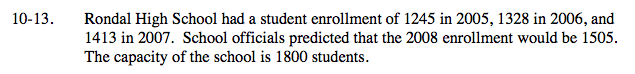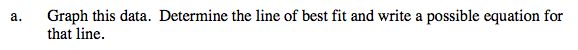To find the line of best fit, either use your graphing calculator or plot the points and draw a line that passes through them.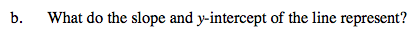The slope represents the number of new students per year. The y-intercept might represent the number of students enrolled when the school opened.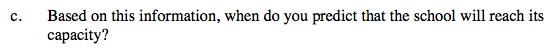Use the line of best fit that you found in part (a) to predict this.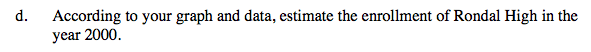See part (c).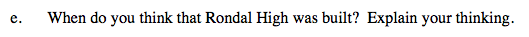The equation will not necessarily help answer this. The school would not have opened with zero students.